﻿ 一种基于混沌与Bit位扰乱的彩色图像加密算法 A Color Image Encryption Algorithm Based on Chaos and Bit Disturbance

Journal of Image and Signal Processing
Vol. 08  No. 01 ( 2019 ), Article ID: 28108 , 9 pages
10.12677/JISP.2019.81003

A Color Image Encryption Algorithm Based on Chaos and Bit Disturbance

Zhiben Zhuang1, Jingyi Liu1, Shiqiang Chen2*

1School of Science, Hubei University for Nationalities, Enshi Hubei

2School of New Materials and Mechanical Engineering, Hubei University for Nationalities, Enshi Hubei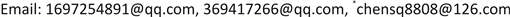Received: Nov. 27th, 2018; accepted: Dec. 13th, 2018; published: Dec. 20th, 2018ABSTRACT

In this paper, we propose a color image encryption algorithm based on improved Henon chaotic map and Bit scrambling. First, we combine the three channels R, G, and B of the plaintext image into a matrix, and also convert it into a binary number. Secondly, the rows and columns of the binary number matrix are disturbed, and the disturbed binary number matrix is restored to a decimal number matrix. Finally, it is divided into three matrices with the same number of pixels, and then the three matrices are subjected to a bitwise XOR operation to obtain the final encrypted image. The experimental results and theoretical analysis demonstrate that the algorithm has a large key space. It can effectively resist the attacks of statistical analysis and gray value analysis, and has a good encryption effect on digital image encryption.

Keywords:Henon Chaotic Map, Bit Scrambling, Color Image Encryption, Bitwise XOR Operation

1湖北民族学院理学院，湖北 恩施

2湖北民族学院新材料与机电工程学院，湖北 恩施Copyright © 2019 by authors and Hans Publishers Inc.1. 引言

2. 相关知识

Henon映射方程的改进

$\left\{\begin{array}{l}x\left(n+1\right)=1+y\left(n\right)-ax{\left(n\right)}^{2}\\ y\left(n+1\right)=bx\left(n\right)\end{array}$ (1)

$a\in \left(0,1.4\right)$$b=0.3$ 时，Henon系统方程处于混沌状态。如图1所示。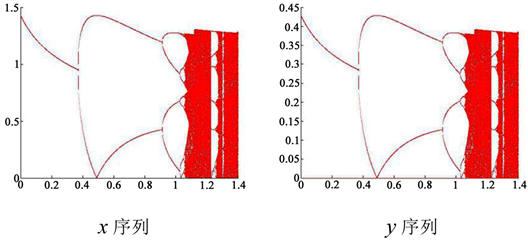Figure 1. Chaotic sequence diagram

$\left\{\begin{array}{l}x\left(n+1\right)=1+ay\left(n\right)-bx{\left(n\right)}^{2}\\ y\left(n+1\right)=cx\left(n\right)-dx{\left(n\right)}^{2}\end{array}$ (2)

$a=0.001,b\in \left(0,2.2\right),c=0.3,d=1.2$ 时，改进后的Henon系统方程处于混沌状态。如图2所示。从图1图2可以看出图2中处于混沌状态的范围更大。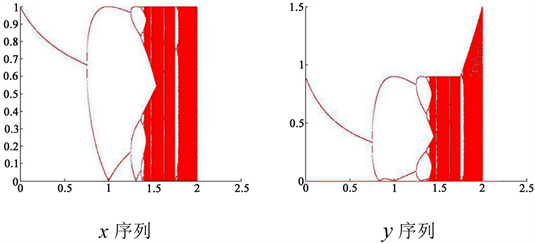Figure 2. Improved chaotic sequence diagram

3. 算法描述

3.1. 加密算法描述

Step 1：把彩色图像 $P$ 的R、G、B三个通道上的矩阵分别取出来，同时把这三个矩阵按行合并成一个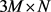的矩阵，记为 $P1$

Step 2：把 $P1$ 转换成一行 $3M×N$ 列的矩阵 $P2$ ，同时把 $P2$ 转换成二进制数矩阵 $P3$

Step 3：输入初始密钥 $a=0.001,b=1.8,c=0.3,d=1.2,x\left(1\right)=0,y\left(1\right)=0$ ；迭代系统(2) $3M×N$ 次，产生两条混沌序列 $x,y$

Step 4：把混沌序列 $x$$y$ 做如下处理：

$H1=mod\left(round\left(x×{10}^{40}\right),7\right)+1$ (3)

 (4)

$y1=mod\left(round\left(abs\left(y\left(4:\left(4+M×N\right)\right)\right)×{10}^{16}\right),256\right)$ (5)

 (6)

Step 5：把 $P3$ 中的每一行二进制数分别进行扰乱，得到矩阵 $P4$ ，扰乱公式如下：

$P4\left(j1,:\right)=circshift\left(P3\left(j1,:\right),H1\left(\left(j1\right),2\right);j1=1,2,\cdots ,3M×N$ (7)

Step 6：把矩阵 $P4$ 的第1列与第2列互换；第3列与第4列互换；第5列与第6列互换；第7列与第8列互换，得到矩阵 $P5$

Step 7：把 $P5$ 转换成十进制数，再把已经转换成十进制数的 $P5$ 转换成1行 $3M×N$ 列的矩阵 $P6$

Step 8：把 $P6\left(1:M×N\right)$$x1$ 进行按位“异或”运算，得到一个新的序列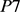，把 $P7$ 转换成 $M×N$ 的矩阵 $P8$

Step 9：把 $P6\left(\left(M×N+1\right):2×M×N\right)$$y1$ 进行按位“异或”运算，得到一个新的序列  ，把 $P9$ 转换成 $M×N$ 的矩阵 $P10$

Step 10：把 $P6\left(\left(2×M×N+1\right):3M×N\right)$$p$ 进行按位“异或”运算，得到一个新的序列 $P11$ ，把 $P11$ 转换成 $M×N$ 的矩阵 $P12$

Step 11：把 $P8$$P10$$P12$ 作如下处理，得到矩阵 $P13$ ，即最终的加密图像， $P13\left(:,:,1\right)=P8$

$P13\left(:,:,2\right)=P10$

$13\left(:,:,3\right)=P12$(8)

3.2. 解密算法的描述

Step 1：把矩阵 $P13$ 作如下处理，得到矩阵 $P14$$P15$$P16$

$P14=P13\left(:,:,1\right)$

$P15=P13\left(:,:,2\right)$

$P16=P13\left(:,:,3\right)$(9)

Step 2：输入初始密钥 $a=0.001,b=1.8,c=0.3,d=1.2,x\left(1\right)=0,y\left(1\right)=0$ ，迭代系统(2) $3M×N$ 次，产生两条混沌序列 

Step 3：把混沌序列 $x$ 做如下处理：

$H1=mod\left(round\left(x×{10}^{40}\right),7\right)+1$ (10)

$x1=mod\left(round\left(abs\left(x\left(4:\left(4+M×N\right)\right)\right)×{10}^{16}\right),256\right)$ (11)

$y1=mod\left(round\left(abs\left(y\left(4:\left(4+M×N\right)\right)\right)×{10}^{16}\right),256\right)$ (12)

$p=mod\left(round\left(abs\left(y\left(\left(2M×N+1\right):end\right)+x\left(\left(M×N+1\right):2M×N\right)\right)×{10}^{16}\right),256\right)$ (13)

Step 4：把 $H1$ 序列作如下处理：

$H2\left(i\right)=8-H1\left(i\right)$ (14)

Step 5：把 $P16$$P14$ 分别进行按位“异或”运算，得到序列 $P17$$P18$$P19$ ，再把序列 $P17$$P18$$P19$ 做如下处理，得到序列 $P20$

$P20\left(1:M×N\right)=P19$

$P20\left(\left(M×N+1\right):2×M×N\right)=P18$

 (15)

Step 6：把 $P20$ 转换成二进制数，同时把已经转换成二进制数的 $P20$ 的列和行还原，得到矩阵 $P21$

Step 7：把 $P21$ 转换成十进制数，并且转换成 $3M×N$ 的矩阵 

Step 8：把 $P22$ 做如下处理，得到矩阵 $P23$ ，解密图像 $P$

$P23\left(:,:,1\right)=P22\left(1:M,:\right)$

$P23\left(:,:,2\right)=P22\left(\left(M+1\right):2M,:\right)$

$P23\left(:,:,3\right)=P22\left(\left(2M+1\right):3M,:\right)$(16)

4. 实验结果

4.1. 实验平台

PC机配置：Intel (R) Core (TM) i3-4170 CPU @ 3.70 GHz 3.70 GHz，内存4 GB，Windows7 32位操作系统。通过Matlab R2014a编写程序实现上述加密算法。

4.2. 实验结果

5. 安全性分析

5.1. 密钥空间分析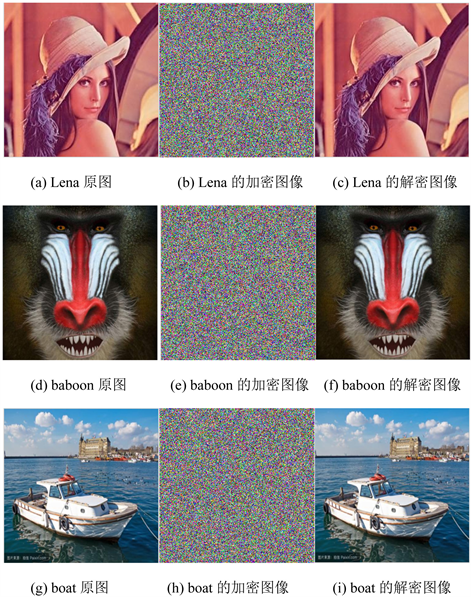Figure 3. Plain text, encrypted image and decrypted imageTable 1. Comparison of key space between this algorithm and other algorithms

5.2. 直方图分析

5.3. 信息熵分析

$H\left(m\right)=-\sum _{i=1}^{L}p\left({m}_{i}\right){\mathrm{log}}_{2}p\left({m}_{i}\right)$ (17)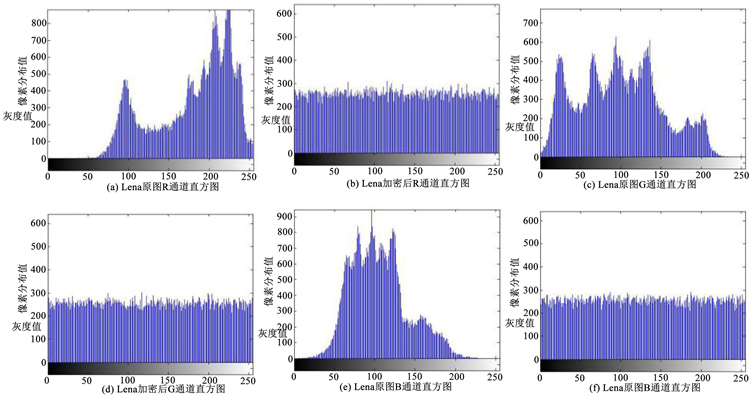Figure 4. Lena’s original image R, G, B three-channel histogram and the encrypted image R, G, B three-channel histogramTable 2. Entropy analysis table for plaintext and encrypted images

5.4. 不动点比和灰度平均变化值分析

$BD\left(G,C\right)=\frac{\sum _{i=1}^{M}\sum _{j=1}^{N}f\left(i,j\right)}{MN}×100%$ ，其中 $f\left(i,j\right)=\left\{\begin{array}{l}1,{g}_{ij}={c}_{ij}\\ 0,{g}_{ij}\ne {c}_{ij}\end{array}$ (18)Table 3. Fixed point ratio analysis of encrypted images

$GAVE\left(C,G\right)=\frac{\sum _{i=1}^{M}\sum _{j=1}^{N}|{c}_{ij}-{g}_{ij}|}{MN}$ (19)Table 4. Analysis of average change of grayscale

6. 结束语

A Color Image Encryption Algorithm Based on Chaos and Bit Disturbance[J]. 图像与信号处理, 2019, 08(01): 15-23. https://doi.org/10.12677/JISP.2019.81003

1. 1. Sun, S. (2018) A Novel Hyperchaotic Image Encryption Scheme Based on DNA Encoding, Pixel-Level Scrambling and Bit-Level Scrambling. IEEE Photonics Journal, 10.

2. 2. Rui, L. (2015) New Algorithm for Color Image Encryption Using Improved 1D Logistic Chaotic Map. Open Cybernetics & Systemics Journal, 9, 210-216.
https://doi.org/10.2174/1874110X01509010210

3. 3. Chai, X., Gan, Z. and Zhang, M. (2017) A Fast Cha-os-Based Image Encryption Scheme with a Novel Plain Image-Related Swapping Block Permutation and Block Diffusion. Multimedia Tools and Applications, 76, 1-25.
https://doi.org/10.1007/s11042-016-3858-4

4. 4. Ahmad, J., Khan, M.A., Ahmed, F., et al. (2017) A Novel Image Encryption Scheme Based on Orthogonal Matrix, Skew Tent Map, and XOR Operation. Neural Computing and Applications.

5. 5. Gao, T. and Chen, Z. (2008) Image Encryption Based on a New Total Shuffling Algorithm. Chaos Solitons& Fractals, 38, 213-220.
https://doi.org/10.1016/j.chaos.2006.11.009

6. 6. Pareek, N.K., Patidar, V. and Sud, K.K. (2006) Image encryption Using Chaotic Logistic Map. Image & Vision Computing, 24, 926-934.
https://doi.org/10.1016/j.imavis.2006.02.021

7. 7. Ahmad, J. and Hwang, S.O. (2015) Chaos-Based Diffusion for Highly Autocorrelated Data in Encryption Algorithms. Nonlinear Dynamics, 82, 1-12.
https://doi.org/10.1007/s11071-015-2281-0

8. 8. Jiang, S., Wang, G. and Jin, P. (2017) A New Image Encryption Algorithm Based on Improved Henon Mapping. Journal of Hangzhou Dianzi University.

9. 9. Guo, Y., Shao, L.P. and Yang, L. (2015) Bit-Level Image Encryption Algorithm Based on Josephus and Henon Chaotic Map. Application Re-search of Computers.

10. 10. Sekertekin, Y. and Atan, Ö. (2017) An Image Encryption Algorithm Using Ikeda and Henon Chaotic Maps. Telecommunications Forum, IEEE, 1-4.

11. 11. Su, Y., Tang, C., Chen, X., et al. (2017) Cascaded Fresnel Holographic Image Encryption Scheme Based on Aconstrained Optimization Algorithm and Henon Map. Optics & Lasers in Engineering, 88, 20-27.
https://doi.org/10.1016/j.optlaseng.2016.07.012

12. 12. Gong, H.L. (2010) Image Encryption Algorithm Based on Henon Map and High Dimensional Matrix Transformation. Microcomputer Information, 26, 87-89.

13. 13. Yue, H.H., Tao, L.I. and Shi, L. (2011) Improved Image Encryption Algorithm Based on Henon Hyperchaotic System. Journal of Computer Applications, 31, 1909-1905.

14. 14. Abdullah, M., Rehab, F. and Ali, M. (2015) Chaos Image Encryption based on DCT Transforms and Henon Map. International Journal of Computer Applications, 127, 1-7.

15. 15. Xie, G.B., Wang, T. and Computer, F.O. (2016) A Novel Hyperchaotic Image Encryption Algorithm Based on Bit Scrambling. Microelectronics & Computer.

16. 16. Changsha (2013) Color Image Encryption Algorithm Based on 2D Logistic Chaotic Map and Bit Rearrange. Computer Science, 40, 300-299.

17. 17. Li, Y., Wang, C. and Chen, H. (2017) A Hy-per-Chaos-Based Image Encryption Algorithm Using Pixel-Level Permutation and Bit-Level Permutation. Optics & Lasers in Engineering, 90, 238-246.
https://doi.org/10.1016/j.optlaseng.2016.10.020

18. 18. Liu, J., Yang, D., Zhou, H., et al. (2018) A Digital Image Encryption Algorithm Based on Bit-Planes and an Improved Logistic Map. Multimedia Tools & Applications, 77, 10217-10233.
https://doi.org/10.1007/s11042-017-5406-2

19. 19. Teng, L., Wang, X. and Meng, J. (2017) A Cha-otic Color Image Encryption Using Integrated Bit-Level Permutation. Multimedia Tools & Applications, 77, 1-14.

20. 20. Zhang, X., Han, F. and Niu, Y. (2017) Chaotic Image Encryption Algorithm Based on Bit Permutation and Dynamic DNA Encoding. Computational Intelligence and Neuroscience, 2017, Article ID: 6919675.
https://doi.org/10.1155/2017/6919675

21. 21. Cao, C., Sun, K. and Liu, W. (2017) A Novel Bit-Level Image En-cryption Algorithm Based on 2D-LICM Hyperchaoticmap. Signal Processing, 143, 122-133.

22. 22. Enayatifar, R., Ab-dullah, A.H., Isnin, I.F., Altameem, A. and Lee, M. (2017) Image Encryption Using a Synchronous Permutation Diffu-sion Technique. Optics and Lasers in Engineering, 90, 146-154.
https://doi.org/10.1016/j.optlaseng.2016.10.006

23. 23. Liu, H., Wang, X., et al. (2012) Image Encryption Using DNA Complementary Rule and Chaotic Maps. Applied Soft Computing, 12, 1457-1466.
https://doi.org/10.1016/j.asoc.2012.01.016

24. NOTES

*通讯作者。